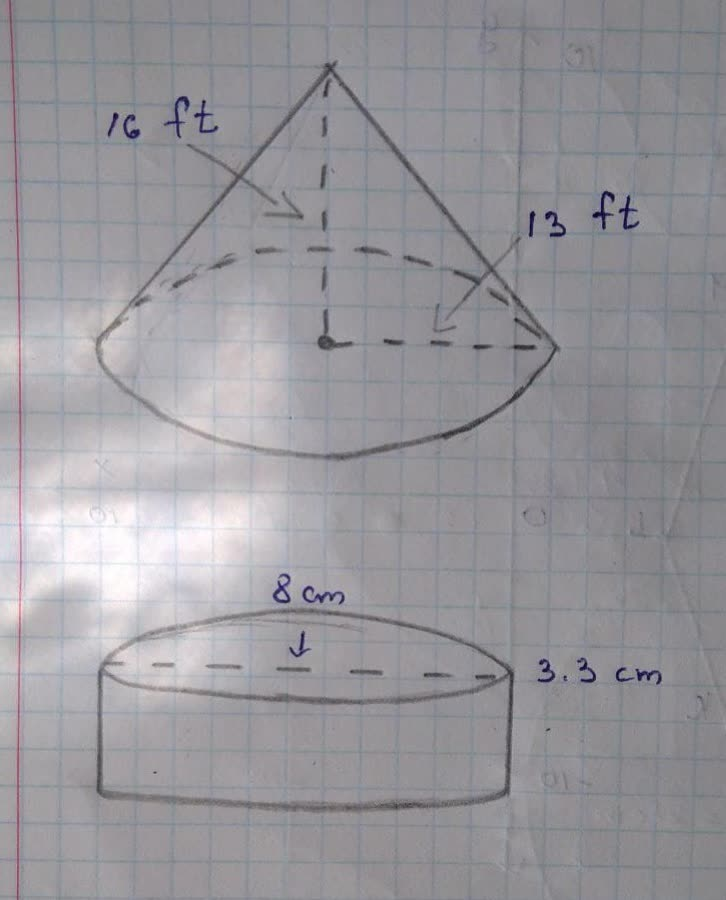What is the volume of this cone? Use \pi \approx 3.14Tomasettiq3 2021-11-29 Answered
What is the volume of this cone?
Use $$\displaystyle\pi\approx{3.14}$$ and round your answer to the nearest hundredth.
What is the volume of this cylinder?
Use $$\displaystyle\pi\approx{3.14}$$ and round your answer to the nearest hundredth.• Questions are typically answered in as fast as 30 minutes

Solve your problem for the price of one coffee

• Math expert for every subject
• Pay only if we can solve itMomp1989
Volume of the cone $$\displaystyle{v}={\frac{{{1}}}{{{3}}}}\pi{r}^{{{2}}}{h}$$
$$\displaystyle{r}={13}{f}{t}$$
$$\displaystyle{h}={16}{f}{t}$$
$$\displaystyle{v}={\frac{{{1}}}{{{3}}}}\times{\left({3.14}\right)}\times{\left({13}\right)}^{{{2}}}\times{\left({16}\right)}$$
$$\displaystyle{v}={2830.19}$$ cubic ft
volume of the cylinder $$\displaystyle=\pi{r}^{{{2}}}{h}$$
$$\displaystyle{r}={\frac{{{d}{i}{a}{m}{e}{t}{e}{r}}}{{{2}}}}={\frac{{{8}{c}{m}}}{{{2}}}}={4}{c}{m}$$
$$\displaystyle{h}={3.3}{c}{m}$$
$$\displaystyle{v}={\left({3.14}\right)}{\left({4}\right)}^{{{2}}}{\left({3.3}\right)}$$
$$\displaystyle={165.79}$$ cubic cm